## How to solve large sparse systems of linear equati...

I have some large systems of linear equations.  The solutions are probability generating functions.  I can get solutions in a few minutes for systems of up to n= 200 eqns or so, but Maple just cycles indefinitely if I try to solve much larger systems.  I really only need to perform Gaussian Elimination, as I only need to solve for one of the n solutions.  The matrices are sparse, there are only 3 non-zero entries per row.  I tried to get help from the manuals but I get the impression that sparse solutions are only available for numeric computations.   Doesn't Maple allow for sparse symbolic solutions?  If so, how to do it?

## How do I calculus the K constant in Einstein's equ...

How can I solve Einstein’s equation and calculus of the value of the K constant in Einstein's equation and the value of the tensor stress energy that fits in this equation?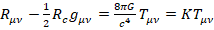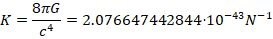After a long hiatus I have come back to the issue of null tetrads in the physics package in light of the updates to  Maple in 2021. I have uploaded a document file to illustrate. See below. My first question concerns the labelling of elements of a null tetrad. After calling the metric 27,37 from Stephani et al, and using Setup to specify a null tetrad, Maple's choice is such that the elements labelled m and bar m are not complex. Rather, both these elements are in fact real, while the elements lablled l and n are complex, with one being the negative complex conjugate of the other. While these are just labels, they don't agree with the usual conventions for the Newman-Penrose formalism, which is disorienting. What convention is Maple using to label the elments of a null tetrad?

Next, I try to specify the null tetrad used by Stephani et al., first by converting it into covariant form (which I did by hand rather than in Maple). In Maple's default null tetrad, the order in which Maple listed the elements of the null tetrad is n, m, bar m, l (as rows in the matrix display for e_[ ]), so I followed that convention (in the conventions of Stephani et al., the first and fourh element should have scalar product -1, the second and third scalar product 1, and all other scalar products zero, which is the case). After entering the matrix and using Setup to specify the null tetrad by the matrix, I get an error message saying that the components of the metric with respect to my tetrad are not just 0, 1, and -1. Yet,  executing eta_[ ]  does not confirm this warning; nor does a computation by hand.

Finally, IsTetrad asserts the tetrad is not null, contrary to the fact that it is a null tetrad.

Since I have followed the conventions implicit in Maple's default null tetrad for this metric, I am puzzled as to what has gone wrong.SKMHH27_37_2021_New.mw

On the other hand, taking into account how Maple 2019 orders the coordinates in Stephani et al 27.37 and labels the null vectors in a null ttetrad, if I translate accordingly what I have in the 2021 Maple file, Maple 2019 confirms Stephani et al.'s null tetrad is indeed a null tetrad, as one would expect. See the following file.SKMHH27_37_2019_Var.mw.

## How can the functional derivative/variation of the...

Hello Everyone,

My questions is about a straight forward as my title suggests. I know how to compute the variation by hand and of course it is in plently of books, but I would like to know how I could possibly do it in Maple as I have some terms with non-trivial contributions where I infact need that specific variation.

I have attached my file I have been working with that has more comments.

RicciAction.mw

Thank you

## How can I Simplify in the physics package a tensor...

Hi MaplePrimes,

I am interested in obtaining some gravitational field equations from an action using the FunDiff command. I have been able to write what I think is a pretty short and quick worksheet(with an arbitrary metric) and I am easily able to obtain the Einstein Field Equations. However, when I introduce some new more complicated terms into the action and apply the Simplify command maple does not appear to be able to evaluate and I end up halting the computation. When I specify a metric Maple, for example Schwarzschild, Maple will easily be able to Simplify my expression but it will use metric components during the process. Where what I am interested in is just the Tensor expression with respective indices.

I was wondering if anyone had any thoughts on how I could resolve this.

I have attached the worksheet that I am working with. I look forward to your thoughts/comments.

Thank you.

ActionFieldEquations.mw

## Copying output together vs seperately...

So I have a problem when copying multiple output from Maple to Word. I am using a simple example here.

When I copy each part of the output as an image SEPERATELY, it comes out much cleaner than if I highlight everything together and then copy. Is there a reason this is the case and is there a way to fix this problem?

Here is the Maple Code:

with(plottools);
with(plots);
ttt1 := textplot([0, 1, "3  +  5  =  __", color = "black", font = ["Arial", "bold", 120]]);
display(ttt1, size = [1000, 200], axes = none);
ttt1 := textplot([0, 1, "1  +  3  =  __", color = "black", font = ["Arial", "bold", 120]]);
display(ttt1, size = [1000, 200], axes = none);

I also uploaded the Maple Worksheet

Here is the output:There are TWO imges in the output in this example. I tried copying both together and pasting them, and then I tried copying them individually. The output is very different in quality.The one copied alot is much cleaner once copied and pasted in Word. Does anyone why this is happening? I don't want to have to copy images individally for obvoius reasons. I know this example is only two images but what if there was alot more, etc.

Thank you

 >>>## Problem with Solving a PDE...

Hi Everyone,

I intend to find the invariants of a 2nd-order Tensor using the representation of the rotational group as an Infinitesimal generator. However, maple does not seem to find the correct invariants $\tr \mathbf{a}$, $\tr \mathbf{a}^2$, $\tr \mathbf{a}^3$. Maple only returns an empty set. I can't seem to figure out why that is the case.

Here is my code>>>>>(1)
 >(2)
 >(3)
 >>(4)Any input would be appreciated!

## Make my Own Code and Call to other file and Get Re...

Hello;

Hope you are fine. I need to learn how can I call my code in the other file of maple, define new value for A and can get results. For this purpose, I just take an simple example as

questionmaple.mw

 >(1)## Syrup Spice Toolbox - Netlists with arbitary volta...

Good day,

I am looking for some guidance with the Syrup toolbox

I have a netlist that was generated with the Altium designer Spice simulator (XSpice based), I was able to import it into LTSpice and verrify the results.

I want to move it into maple to make use of the modellica code generation.

I then began modifying the netlist in order to meet the netlist syntax of Syrup. One of the most prominant change I needed to make was the arbitrary input nodes for my Voltage dependant Voltage sources.

Therefore, for any line that implies arbitrary sources such as:

E_U8_E1 U8_N208620 LO
+ VALUE { IF(V(U8_N208706, 0) > 0.5, 5, -5) }

was changed to:

V1    16   17 1vdc

E_U8_E1 U8_N208620 LO 16 17
+ VALUE { IF(V(U8_N208706, 0) > 0.5, 5, -5) }

This, of course, is a non ideal workaround which brings me to question 1,

Is there a way to define a arbitrary source with the Spice parser in Syrup?

Once these changes were made, it seems the Solve function in Syrup can parse the netlist without issue, however when it gets to the Solve portion it throws the following error:

Solve("file://C:/Users/msavoy/Documents/Spice_cct/inverter_newfet_nonot.cir", 'tran');
Solve: Analyzing SPICE deck "The Apple" (ignoring this line)
Error, (in Syrup:-Solve) invalid input: rcopy uses a 1st argument, A (of type anything), which is missing


Attached is a copy of the netlist, inverter_newfet_nonot.txtI am honestly at a lost as to what could be throwing the rcopy error

Furthermore, I wanted to test out the Modellica code generation in Syurp, it appears that it does not like having subcircuits, as running the test:

V 1 0 1
L1 1 2 L
C1 2 0 C
L2 2 3 L
C2 3 0 C
L3 3 4 L
C3 4 0 C
Vshort 4 5 0
Rt 5 0 1
.SUBCKT TEST A B C Y
V1 14 15 1
EINT YINT 0 14 15
+ VALUE {IF(V(A) > 0.5 && V(B) > 0.5 && V(C) > 0.5, 1, 0)}
RINT YINT Y 1
CINT Y 0 1n
.ENDS TEST
.end

provides the error of:

test_c := ToModelica("file://C:/Users/msavoy/Documents/Spice_cct/test_cct_RCL.txt", 'probes' = ["Rt.v"], 'parameters' = {C = 2, L = 1});
Error, (in Syrup:-ToModelica) invalid input: nodeToModelica expects its 1st argument, node, to be of type nonnegint, but received Y


Is it possible to define a sub circuit in a netlist that is to be converted to Modellica, or do all subcircuits need to be defined in their own file?

Thank you

## How to compute the restricted-edge-connectivity of...

！Edits: I have found  a existing polynomial algorithm, but I still have difficulty implementing it. 2022/10/26

The edge connectivity of a connected graph G  is the minimum number of edges whose deletion from the graph G disconnects G. Below we are concerned with a particular kind of edge-cut.

• For a connected graph G=(V ,E), an edge set S ⊂ E is a restricted-edge-cut, if G−S is disconnected and every connected component of  G−S has at least 2 vertices.

Clearly, a restricted-edge-cut is an edge cut with a special requirement.

• The restricted-edge-connectivity of G, denoted by κres(G), is defined as the cardinality of a minimum restricted-edge-cut.

For example, a graph g is as follows.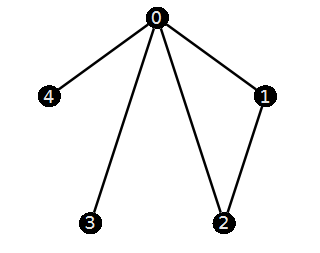Clearly, its edge-connectivity is 1 since (0,3) or (0,4) is a edge cut of g. But we can find that  if we remove the edge (0,3), then "3" is a isolated vertex. Similarly, "4" is a isolated vertex if we remove  (0,4). It is not difficult to find g has exactly the two cut-edges (0,3) and (0,4).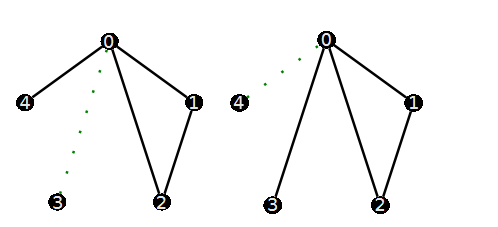Based on the definition of the restricted-edge-cuts, neither {(0,3)} nor  {(0,4)} are restricted-edge-cuts A minimum restricted-edge-cut is {(0,1),(0,2)} since every connected component of G-{(0,1),(0,2)} has  (at least) 2 vertices.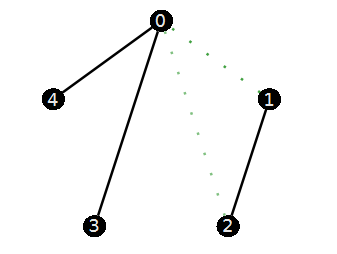So  κres(g) is 2.  My problem is:

Given a graph G, how to calculate the restricted-edge-connectivity of  G?  Moreover, how to find a minimum restricted-edge-cut?

A specific graph that I want to test is as follows. (it has 16 vertices and 56 edges.) I would like to calculate its restricted-edge-connectivity and find a minimum restricted-edge-cut.

g:=ConvertGraph("O~tIID@wL~jPbOqgLJ@p");
vertexcolor="Black",edgethickness=2])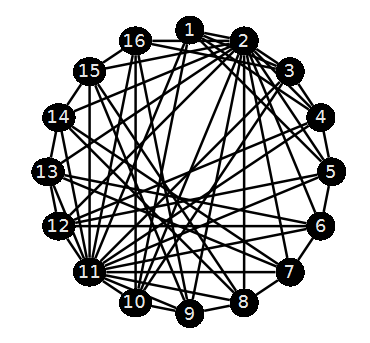EdgeConnectivity(g) 

6

One option I came up with is to find all 6-edge subsets first. Test if they satisfy  the restricted condition (one by one). Then continue to increase to 7 or more. But this violent calculation may get stuck in the first step. That is, test all the minimum edge cut sets (Note that we will consider 32468436 edge-subsets!) I was referring to the efficiency aspect.

with(Iterator):
C:=Combination(58,6):
K:=Edges(g):
#sub:=seq( K[[seq(c[]+1)]], c=C); # do not run this code since it has 32468436 members.

Any language is acceptable.( C or C++, Python. )

PS: Some time ago, I also asked a related question (but with some differences) on mathematica stack （Find all the minimum edge cuts of a graph）. Although Bob Hanlon  gave a reply, the actual result is not good.

Edits: The following literature gives a polynomial algorithm for computing the restricted-edge-connectivity of a given  graph. The heart of it is to computing the least cardinality of some  edge-pairs's edge separator. I'm stuck here.

• Esfahanian A H, Hakimi S L. On computing a conditional edge-connectivity of a graph[J]. Information processing letters, 1988, 27(4): 195-199.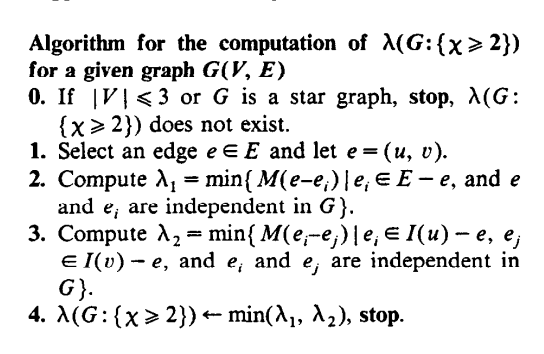How to implement this algorithm is my current concern.

## Why the polysols does not work?...

polysols(diff(u(x), x) = u(x)^2 - 1) produces no results, while it can be verified by direct observation that u(x) = 1 is a polynomial solution.

## Error, MapleSim does not evaluate to a module...

I have maplesim installed and I get the error "Error, MapleSim` does not evaluate to a module" when I run A:=MapleSim:-LinkModel(); in a Maple worksheet.

Does anyone know how to solve this error?

## Why can't Maple solve the below code for some par...

Hello everybody.

I wrote the attached code. this code gives a good result when "H" parameter is equal to 0.5. Even in this condition if I change other parameters, I can get a true result as well. But when I change "H" parameter to 0.6,0.7,0.8 or 0.9 the code can't be executed anymore and I can't get a result.

based on the paper, for H=0.6,0.7,0.8 or 0.9. we have a result.

what's the problem?

1.mw

## Font-size of caption, labels and axes on plots sma...

Maple 2021.
I expect after exporting to pdf view of page with plot like on fig. 1.It means with font=[TIMES, ROMAN, 12] as in maine text.
But I obtaned this view of page on pdf (fig. 2) with smaller font-size.In previouse versions there are no the problem like this.
Dear community, how to fix this annoying inconvenience?fig. 1.fig. 2.

## Maple Bombs While Entering Text...

 1 2 3 4 5 6 7Last Page 1 of 33